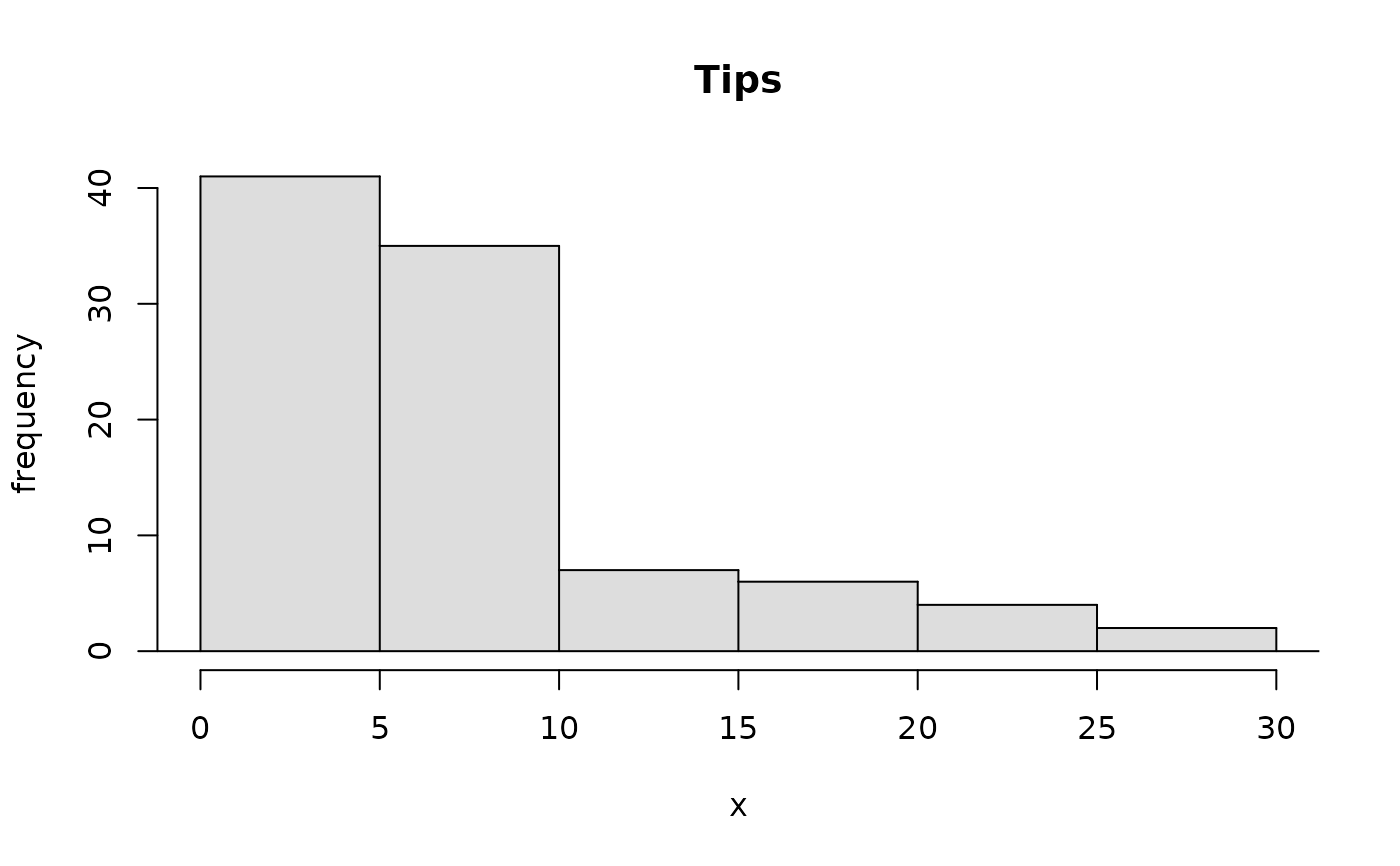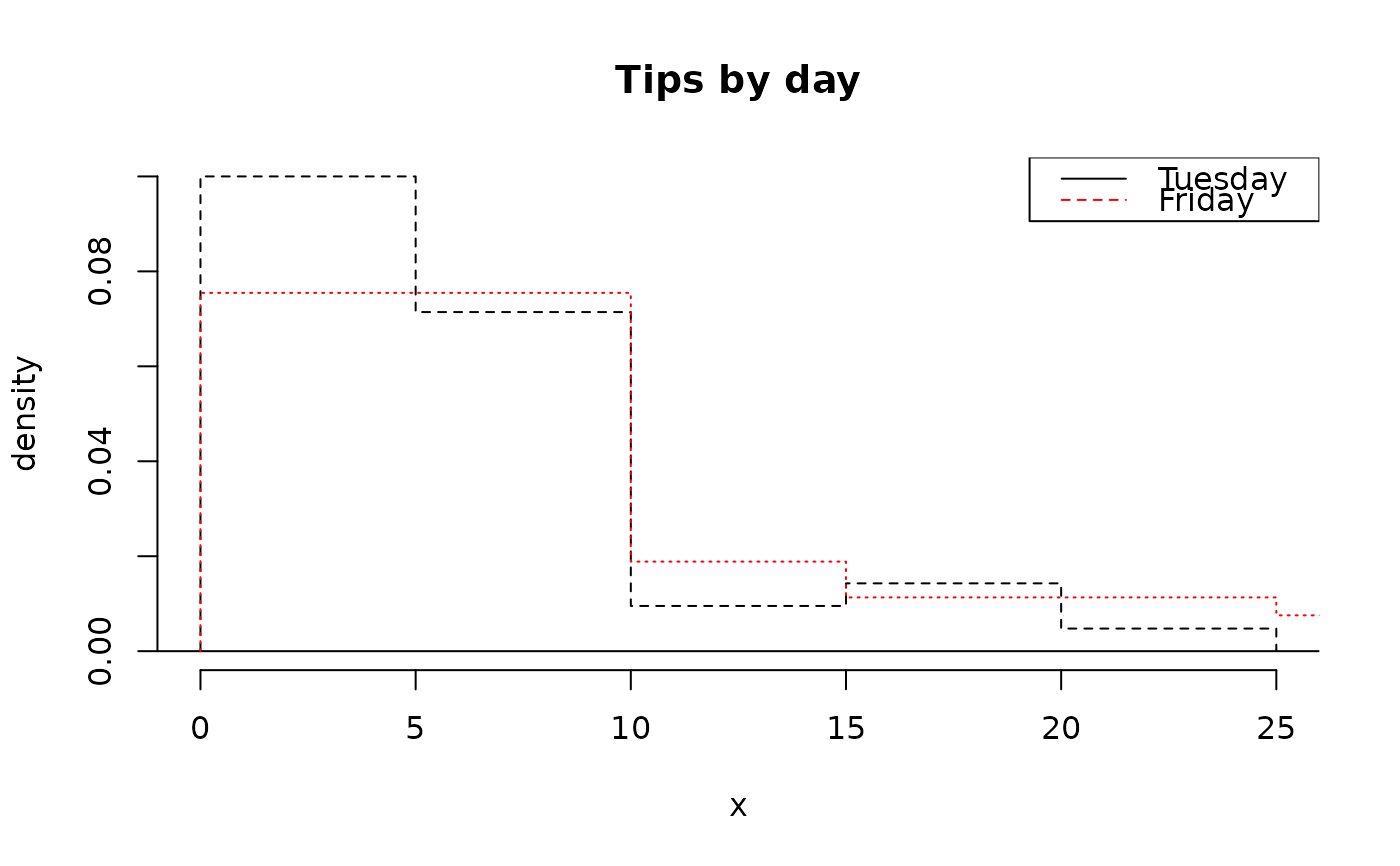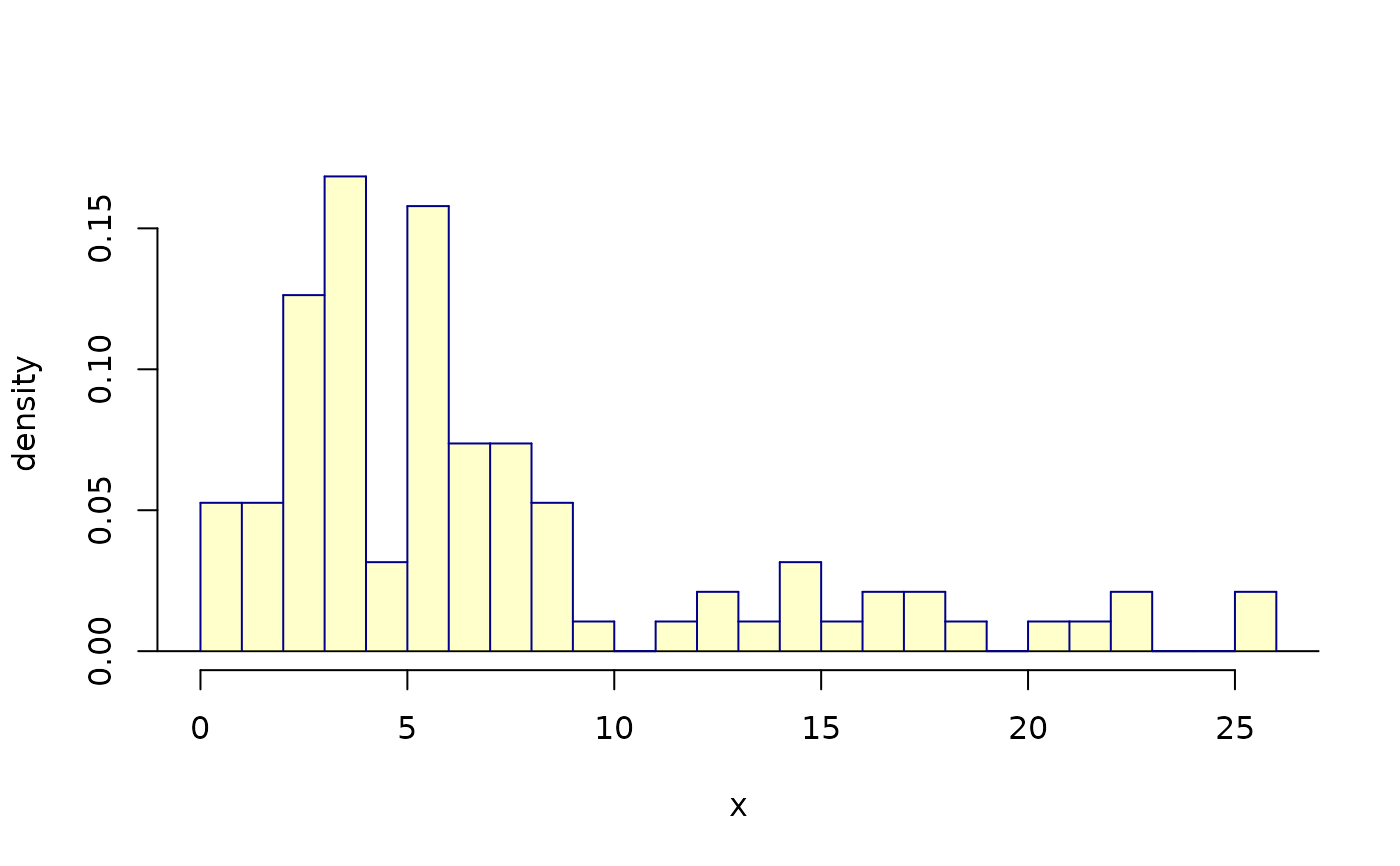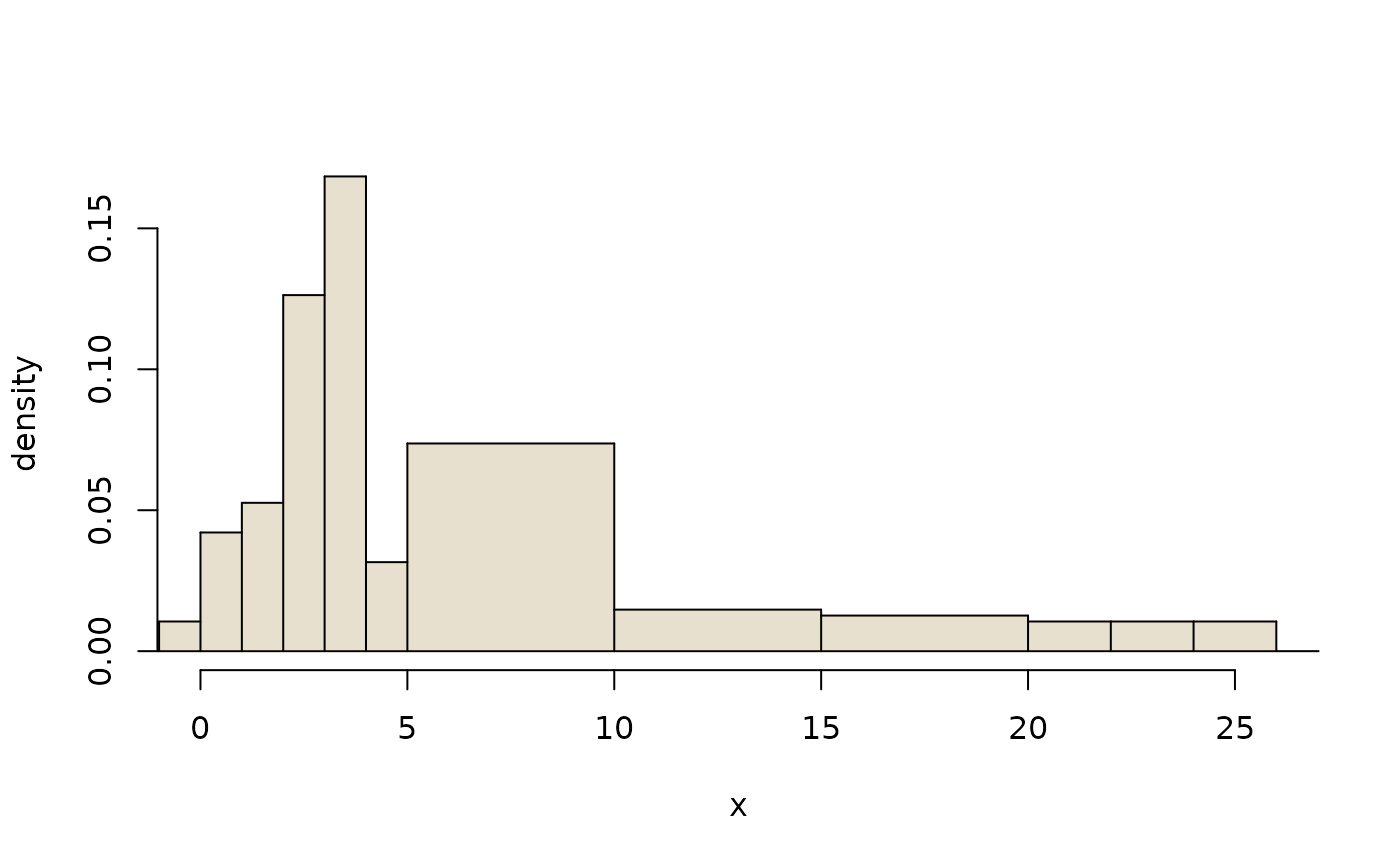Create histograms and hollow histograms. This function permits easy color and appearance customization.

## Usage

histPlot(
x,
border = "black",
breaks = "default",
probability = FALSE,
hollow = FALSE,
lty = 2,
lwd = 1,
freqTable = FALSE,
right = TRUE,
axes = TRUE,
xlab = NULL,
ylab = NULL,
xlim = NULL,
ylim = NULL,
...
)

## Arguments

x

Numerical vector or a frequency table (matrix) where the first column represents the observed values and the second column the frequencies. See also freqTable argument.

col

border

Color of histogram bin borders.

breaks

A vector for the bin boundaries or an approximate number of bins.

probability

If FALSE, the frequency is plotted. If TRUE, then a probability density.

hollow

If TRUE, a hollow histogram will be created.

If TRUE, the histogram is added to the plot.

lty

Line type. Applies only if hollow=TRUE.

lwd

Line width. Applies only if hollow=TRUE.

freqTable

Set to TRUE if x is a frequency table.

right

Set to FALSE to assign values of x that fall on a bin margin to the left bin. Otherwise the ties default to the right bin.

axes

If FALSE, the axes are not plotted.

xlab

Label for the x axis.

ylab

Label for the y axis.

xlim

Limits for the x axis.

ylim

Limits for the y axis.

...

Additional arguments to plot. If add is TRUE, these arguments are ignored.

boxPlot, dotPlot, densityPlot

David Diez

## Examples



histPlot(tips$tip, main = "Tips")# overlaid hollow histograms histPlot(tips$tip[tips$day == "Tuesday"], probability = TRUE, hollow = TRUE, main = "Tips by day" ) histPlot(tips$tip[tips$day == "Friday"], probability = TRUE, hollow = TRUE, add = TRUE, lty = 3, border = "red" ) legend("topright", col = c("black", "red"), lty = 1:2, legend = c("Tuesday", "Friday") )# breaks and colors histPlot(tips$tip,
border = "darkblue",
probability = TRUE,
breaks = 30,
lwd = 3
)# custom breaks
brks <- c(-1, 0, 1, 2, 3, 4, seq(5, 20, 5), 22, 24, 26)
histPlot(tips\$tip,
probability = TRUE,
breaks = brks,